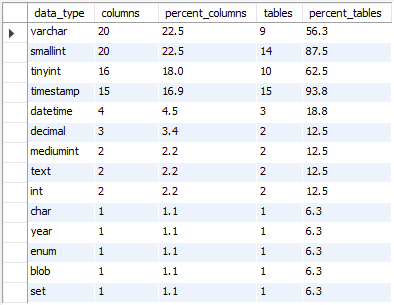## Find most used data type in MySQL database

Article for:

The query below returns data types used in a database (schema) ordered by the number of their occurrence.

## Query

``````select data_type,
count(*) as columns,
cast(100*count(*)/sum_all.columns as decimal(36,2))
as percent_columns,
count(distinct concat(col.table_schema, '.', col.table_name))
as tables,
cast(100*count(distinct concat(col.table_schema,'.',col.table_name))
/ sum_all.tables as decimal(36,2)) as percent_tables
from information_schema.columns col
join (select count(distinct concat(c.table_schema, '.', c.table_name))
as tables,
count(*) as columns
from information_schema.columns c
join information_schema.tables t
on c.table_schema = t.table_schema
and c.table_name = t.table_name
where t.table_schema not in ('information_schema', 'mysql',
'performance_schema', 'sys')
and t.table_type = 'BASE TABLE'
) sum_all on true
join information_schema.tables tab
on col.table_schema = tab.table_schema
and col.table_name = tab.table_name
where tab.table_schema not in ('information_schema', 'mysql',
'performance_schema', 'sys')
and tab.table_type = 'BASE TABLE'
group by data_type,
sum_all.columns,
sum_all.tables
order by columns desc;
``````

## Columns

• data_type - built-in or user data type without length or precision, e.g. int, varchar or datetime
• columns - number of columns in a database (schema) with this data type
• percent_columns - percentage of columns with this data type. The rows total 100%
• tables - number of tables in a database (schema) with this data type
• percent_tables - percentage of tables with columns that have this data type.

## Rows

• One row represents one data type
• Scope of rows: all data types used in a database (schema)
• Ordered by number of columns in descending order

## Sample results

Sample results for the Sakila database (schema). As you can see most popular data type is smallint and varchar - both are used in 20 columns which is 22.5% of all columns in 16 tables.

Note that smallint data type is used in 14 tables that represent 87.5% of all tables, whereas varchar data type is used in 9 tables which is 56.3%.